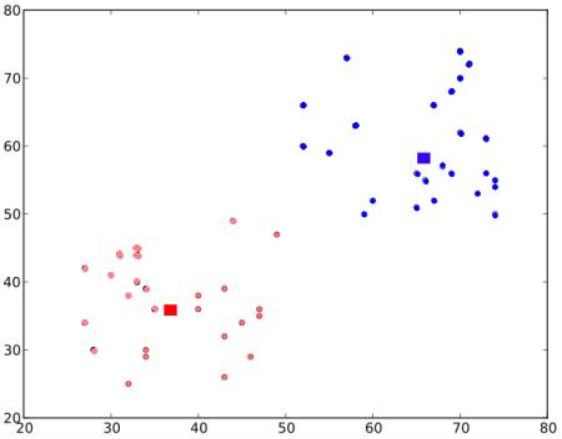### Understanding the Kmean Algorithm(OpenCV)

Objective:

Lesson:
As we are going ahead in time, The usage of Machine learning increases day after day, So, I decided to explain the working of Kmean algorithm. Let us begin.

K Mean is the simplest unsupervised learning algorithms which are used to solve the problem which involves grouping or clustering. The K-means algorithm is used to find clusters or groups which have not been labelled in the data and give us the power to make the decision on grouping formed by this algorithm. Once the algorithm has been initialized, completed its working and clusters are defined, new data points can be easily assigned to the most appropriate cluster.

Let us consider the graph given below,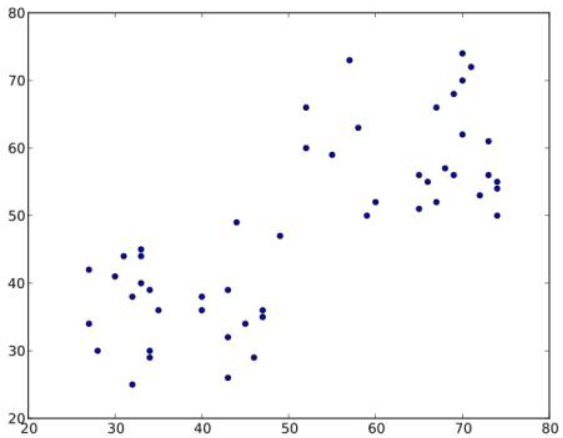It works in the following steps:
1) The algorithm randomly chooses two centroids, C1 and C2.
2) It calculates the distance from each point to both centroids. If the data point is closer to C1, then that data will be labelled as 0. If it is closer to C2, then it will be labelled as 1.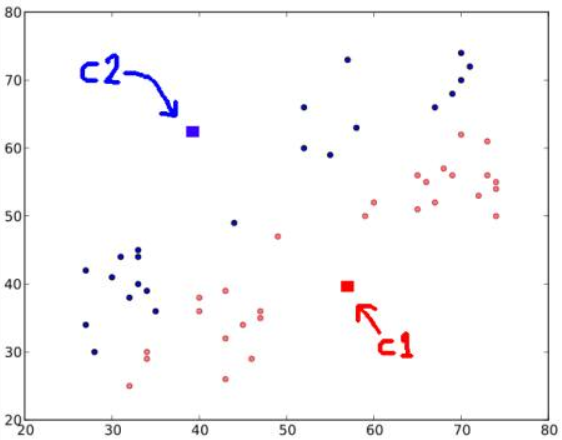3) Now, we calculate the average of all blue points and red points separately and that will be our new centroids. That is C1 and C2 shift to newly calculated centroids.

4) step- 2 and step- 3 will be iterated until both centroids are converged to fixed points.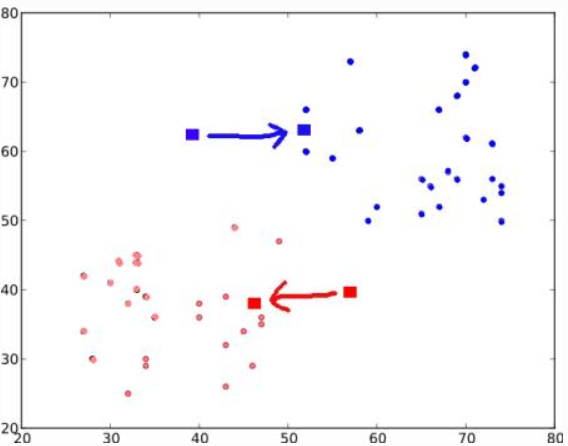These points are such that the sum of distances between test data and their corresponding centroids are minimum. Or simply, the sum of distances between two data point.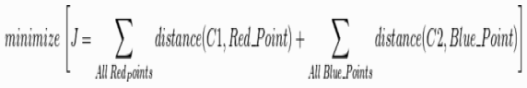5) Finally, we will get a precise point of the centroid.